## Hydrocarbons

Hydrocarbons are organic compounds which are composed of carbon and hydrogen only. They are grouped broadly into three parts:

• Aliphatic hydrocarbons (chain compounds)
• Alicyclic hydrocarbon (these are aliphatic compounds which are in ring structures)
• Aromatic hydrocarbons.

The aliphatic hydrocarbons are further divided into three classes: alkanes, alkenes and alkynes.

## Alkanes

Alkanes are aliphatic hydrocarbons of general molecular formula CnH2n+2. Where n is a whole number whose value starts from 1.

The first and the simplest member is when n is 1. i.e., CH4.

## Homologous Series

A homologous series is a group of organic compounds which follow a regular structural pattern, in which each successive member differs in composition by the addition of a - CH2 group.

The table below shows the first ten alkanes and their formula:

 number of carbon atoms Formula Compound n =1 CH4 Methane n = 2 C2H6 Ethane n = 3 C3H8 Propane n = 4 C4H10 Butane n = 5 C5H12 Pentane n = 6 C6H14 Hexane n = 7 C7H16 Heptane n = 8 C8H18 Octane n= 9 C9H20 Nonane n = 10 C10H22 Decane

Characteristics of Homologous Series:

1. There is a general molecular formula for all members of a particular homologous series , for example, CnH2n+2 for the alkanes.

2. The molecular formula of each member differs from the next by CH2.

3. Members of a homologous series possess similar chemical properties (as a result of their similar molecular structures), though to different degrees.

Example, the chemical reactions of the alkanes include substitution and combustion.

4. Members have different physical properties. For the alkanes, the first four members are gases at ordinary temperature and pressure, while the next five to ten members are liquids.

As the number of carbon atoms increases, solid members are obtained (e.g. C20H42). The boiling and melting points increase with increase in carbon atoms.

Although the alkanes are sparingly soluble in water, their solubility decreases with increase in carbon atoms per molecule.

5. General methods of preparation are known, and can be applied to any member of the series.

6. The names of all members have similar endings. Example, alkanes end with -ane.

7. There is the possibility of each member having its atoms arranged differently to form different structures called isomers. As the number of carbon atoms increases, the number of possible arrangement of those atoms increases rapidly. Example, for the alkanes, there are 2 isomeric butanes, 3 pentanes, and 5 hexanes.

## Structure of Alkanes

In alkanes, example, methane, carbon uses sp3 hybridization, that is, the one 2s orbital is mixed with the three 2p orbitals to form four atomic orbitals of equal energies (i.e. four hybridized orbitals).Hybridized orbitals (sp3)

The four sp3 orbitals then combine separately with the s orbital of four hydrogen atoms to form methane, CH4.

Here, each of the bonds between carbon and hydrogen is sp3 - s and is called sigma bond (σ) (this is the bond between two hybridized orbitals, or between a hybridized orbital and an s orbital).

Note: all available orbitals in the valence shell of carbon are used, hence, the compound formed is saturated.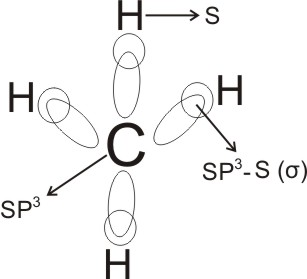Alkanes are therefore tetrahedral in shape - the four covalent bonds are directed to the four corners of a regular tetrahedron, the bond angle is about 109.5o.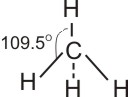Methane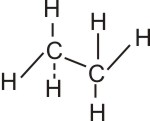Ethane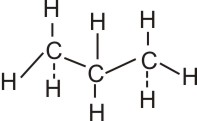Propane

Note:

• The structure of alkanes is not flat as is usually written (for quick writing), but tetrahedral as shown above.
• When carbon atoms form single bonds, they use sp3 hybridization.

Cyclic structures are also possible in alkanes. These are called alicyclic compounds. They are formed when the end carbon atoms of an open aliphatic chain join together, and they have the general formula CnH2n

Example: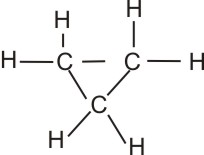Cyclopropane

Since all members of alkanes have similar methods of preparation, as well as similar chemical properties, the simplest member, methane is usually used to represent them.

Related:

Methane
Isomerism in Alkanes
IUPAC Nomenclature of Alkanes
Petroleum and Petrol

 Like This Post? Please Share!!!!!!!!

Copyright , All Rights Reserved Free Chemistry Online | About Us | Usage of Content | Total Disclosures | Privacy Policy